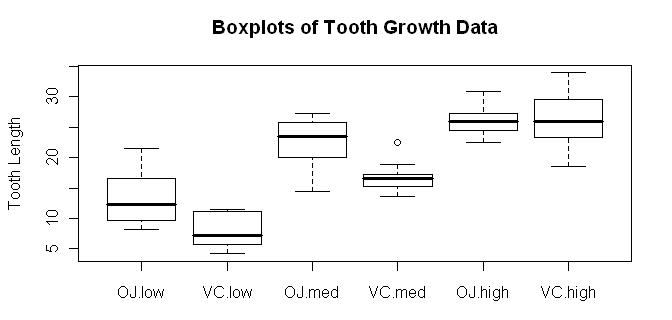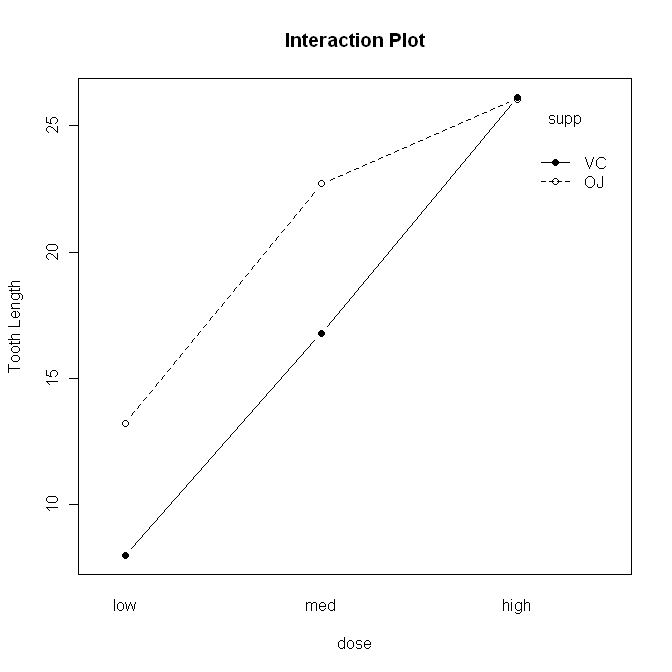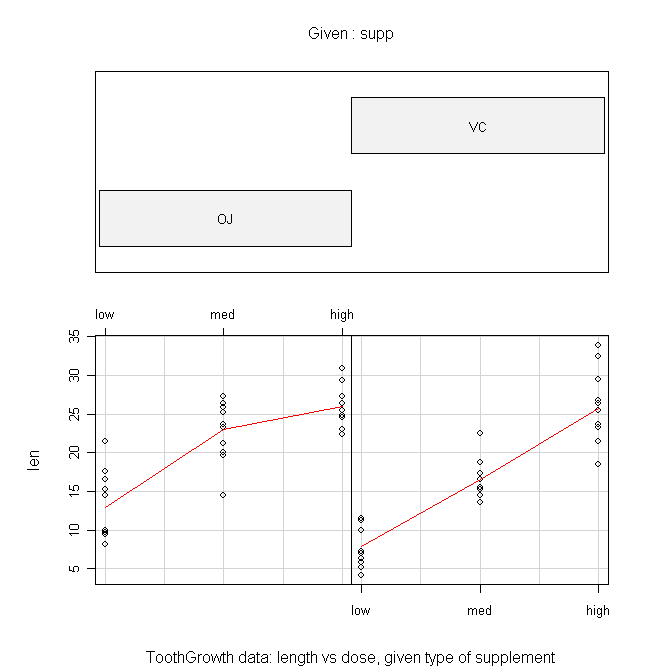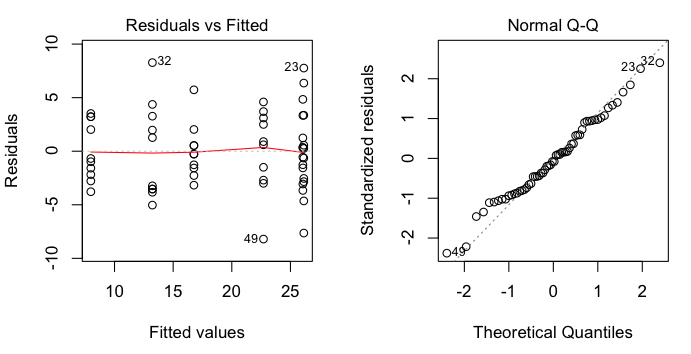| Table of Contents | Function Reference | Function Finder | R Project | FACTORIAL BETWEEN SUBJECTS ANOVA Preliminaries Model Formulae You would probably profit from reading the Model Formulae tutorial before going on. Complexities Anyone who had a graduate school course in analysis of variance is probably still having flashbacks. Experimental designs can become extremely complex, with complications like nesting, blocking, split-plot, Latin square, etc. In this short tutorial, I will stick to the basics, which is to say I will stick to factorial designs (which involve replication in the design cells) that are entirely between subjects and that involve no restrictions on randomization (e.g., such as blocking, etc.). While we're on the topic of complexity, I should add a comment about keeping experimental designs balanced (i.e., equal numbers of subjects in each cell of the design). In single-factor designs, this is not so crucial, as unbalancing the design has the primary effect of making the ANOVA more sensitive to violation of assumptions. However, when a factorial design becomes unbalanced, either because of subject attrition or because the study is observational in nature and subjects were "taken as they came," then the factors lose their independence from one another. BALANCED DESIGNS ONLY will be discussed in this tutorial. Unbalanced Factorial Designs now have a tutorial to themselves. If you have an unbalanced factorial design and are not familiar with the issues involved, READ THAT TUTORIAL before using these procedures. Summarizing Factorial Data For this tuturial, we will examine data on the effect of vitamin C on tooth growth in guinea pigs. ```> data(ToothGrowth) > str(ToothGrowth) 'data.frame': 60 obs. of 3 variables: \$ len : num 4.2 11.5 7.3 5.8 6.4 10 11.2 11.2 5.2 7 ... \$ supp: Factor w/ 2 levels "OJ","VC": 2 2 2 2 2 2 2 2 2 2 ... \$ dose: num 0.5 0.5 0.5 0.5 0.5 0.5 0.5 0.5 0.5 0.5 ... > summary(ToothGrowth) len supp dose Min. : 4.20 OJ:30 Min. :0.500 1st Qu.:13.07 VC:30 1st Qu.:0.500 Median :19.25 Median :1.000 Mean :18.81 Mean :1.167 3rd Qu.:25.27 3rd Qu.:2.000 Max. :33.90 Max. :2.000``` The variables are "len", which is tooth length, the response variable, the way in which the supplement was administered, "supp", of which the levels are "OJ" (as orange juice) and "VC" (as ascorbic acid), and "dose" of the supplement in milligrams (0.5, 1.0, and 2.0). To begin, we should note that R considers "dose" to be numeric. If we want dose treated as a factor (we do), we will have to be absolutely certain that R sees it as such when we construct the model formula. Otherwise, we will end up with an analysis of covariance, which is not what we want. First, let's end all doubt that we want "dose" to be a factor. ```> ToothGrowth.bak = ToothGrowth > ToothGrowth\$dose = factor(ToothGrowth\$dose, + levels=c(0.5,1.0,2.0), + labels=c("low","med","high")) > summary(ToothGrowth) len supp dose Min. : 4.20 OJ:30 low :20 1st Qu.:13.07 VC:30 med :20 Median :19.25 high:20 Mean :18.81 3rd Qu.:25.27 Max. :33.90``` The function factor() is used to declare "ToothGrowth\$dose" as a factor, with levels currently coded as 0.5, 1.0, and 2.0, but now recoded with labels of "low", "med", and "high". Just to be sure, I looked at a summary. Can't be too careful. And be ESPECIALLY CAREFUL with the factor() function. One little typo here can turn your data to garbage. I'd recommend making a backup copy of the data frame before fooling with it like that. Which you'll notice I did. I've learned that the hard way! Now let's check for a balanced design by crosstabulating the factors. Note: You could do it fancier by using replications(len~supp*dose, data=ToothGrowth), but crosstabling the factors is easier and gives a more straightforward answer. ```> with(ToothGrowth, table(supp, dose)) dose supp low med high OJ 10 10 10 VC 10 10 10``` We find that we have 10 subjects (teeth--actually odontoblasts) in each of the 2x3=6 design cells. We have to assume that each of these tooth measurements is an independent measurement. For example, it would be bad news if more than one of these measurements came form the same subject. There are a number of ways the data can be summarized graphically. First, let's look at boxplots. ```> boxplot(len ~ supp * dose, data=ToothGrowth, + ylab="Tooth Length", main="Boxplots of Tooth Growth Data")```Try dose * supp and see how the boxplots change. In the meantime, we should definitely make a note to check our homogeneity and normality assumptions! We might get a better idea of what our main effects and interactions look like if we look at a traditional interaction plot, also called a profile plot. ```> with(ToothGrowth, interaction.plot(x.factor=dose, trace.factor=supp, + response=len, fun=mean, type="b", legend=T, + ylab="Tooth Length", main="Interaction Plot", + pch=c(1,19)))```The syntax is straightforward, but there are a lot of options. First, "x.factor=" specifies the factor to be plotted on the horizontal axis. Second, "trace.factor=" specifies the factor to plotted as individual lines on the graph. Third, "response=" specifies the response variable. Fourth, "fun=" specifies the function to be calculated on the response variable. "Mean" is the default, so we could have left this one out. Fifth, "type=" specifies whether to plot lines, points, or both. We plotted "b"oth. We also asked for a legend, a label on the y-axis, and a main title on the graph. Finally, we specified the point characters to use. We could also have changed the line type, line color, and just about everything else about the graph, if we wanted to get really fussy, er, I mean, aRtistic. Another type of graphic we could look at is called a conditioning plot. I shall borrow the example right off the help page for the ToothGrowth data set. ```> coplot(len ~ dose | supp, data=ToothGrowth, panel=panel.smooth, + xlab="ToothGrowth data: length vs dose, given type of supplement")```The formula is the tricky part here and should be read as "length by dose given supplement." The "panel=" option draws the red lines when set to "panel.smooth". The top panel can be suppressed by setting "show.given=" to FALSE. This gives a much more sensible looking plot in my humble opinion. Numerical summaries of the data can be done with the tapply() function. ```> with(ToothGrowth, tapply(len, list(supp,dose), mean)) low med high OJ 13.23 22.70 26.06 VC 7.98 16.77 26.14 > with(ToothGrowth, tapply(len, list(supp,dose), var)) low med high OJ 19.889 15.295556 7.049333 VC 7.544 6.326778 23.018222``` Remember, in tapply(), the first argument is the response variable, the second argument is a list of factors by which the response should be partitioned (first factor given will be printed in the rows of the resulting table), and the third argument is the function you wish to calculate. Once again, we make note of some consternation over those cell variances. We could also use the by() function (same syntax), but the output is not nearly so concisely formatted. The aggregate() function is tidier, and the function has a much more convenient formula interface, but I still prefer the output of tapply(). ```> aggregate(len ~ supp + dose, ToothGrowth, FUN=mean) supp dose len 1 OJ low 13.23 2 VC low 7.98 3 OJ med 22.70 4 VC med 16.77 5 OJ high 26.06 6 VC high 26.14``` Another way to get similar information is to use the model.tables() function, but the ANOVA has to be done first and saved into a model object. (WARNING: Do not do this with unbalanced data. The answer will NOT be correct!) `> aov.out = aov(len ~ supp * dose, data=ToothGrowth)` Note: The model formula specifies "length as a function of supplement and dose with all possible interactions between the factors. (The asterisk says "include the interactions.") Now we can look at some model tables. ```> model.tables(aov.out, type="means", se=T) Tables of means Grand mean 18.81333 supp supp OJ VC 20.663 16.963 dose dose low med high 10.605 19.735 26.100 supp:dose dose supp low med high OJ 13.23 22.70 26.06 VC 7.98 16.77 26.14 Standard errors for differences of means supp dose supp:dose 0.9376 1.1484 1.6240 replic. 30 20 10``` We have requested the various cell and marginal means along with standard errors for the differences between means. The standard errors are calculated in the usual way for comparisons by protected t-tests (Fisher LSD). The options must be explicitly specified, as they are not the defaults (see the help page). Finally, let's test for homogeneity of variance. The function for the Bartlett test will not properly handle a factorial design, and it won't warn you about it either! It'll give you an answer, but that answer will be wrong. The Fligner-Killeen test function doesn't understand factorial designs either, but at least it warns you. A work-around is to create a factor that identifies every one of the design cells individually. ```> ToothGrowth\$cells = factor(paste(ToothGrowth\$supp, ToothGrowth\$dose, sep=".")) > summary(ToothGrowth) len supp dose cells Min. : 4.20 OJ:30 low :20 OJ.high:10 1st Qu.:13.07 VC:30 med :20 OJ.low :10 Median :19.25 high:20 OJ.med :10 Mean :18.81 VC.high:10 3rd Qu.:25.27 VC.low :10 Max. :33.90 VC.med :10 > bartlett.test(len ~ cells, data=ToothGrowth) Bartlett test of homogeneity of variances data: len by cells Bartlett's K-squared = 6.9273, df = 5, p-value = 0.2261``` Aside: The paste() functions "pastes" together strings. For example: ```> paste("horse", "shoe", sep="")  "horseshoe"``` Here we used it to paste together the level names from the two factors, separated by a period. The default separater is a space, by the way. The leveneTest() function in the "car" package (not installed by default) appears to handle the formula interface properly, although the help page is unclear about this. ```> library(car) # will fail if you haven't installed this package > leveneTest(len ~ dose * supp, data=ToothGrowth) Levene's Test for Homogeneity of Variance (center = median) Df F value Pr(>F) group 5 1.7086 0.1484 54``` The cell variances are not significantly different. Factorial ANOVA We've already run the ANOVA above when we wanted to look at the model tables, but just to remind you, the function was as follows. `> aov.out = aov(len ~ supp * dose, data=ToothGrowth)` You do not have to execute it again if you've already done it. This creates a model object in your workspace, which we've named "aov.out", that contains a wealth of information. The extension to cases with more factors, for completely between-groups designs where no Error structure needs to be specified, would be straightforward. Just list all the factors after the tilde and separate them by asterisks. This gives a model with all possible main effects and interactions. To leave out interactions, separate the factor names with plus signs rather than asterisks. Before we print anything to the Console, I'm going to turn off the printing of significance stars because I find them annoying. `> options(show.signif.stars=F)` Now we can look at the ANOVA table using either summary() or summary.aov(). ```> summary(aov.out) Df Sum Sq Mean Sq F value Pr(>F) supp 1 205.35 205.35 15.572 0.0002312 dose 2 2426.43 1213.22 92.000 < 2.2e-16 supp:dose 2 108.32 54.16 4.107 0.0218603 Residuals 54 712.11 13.19``` We see two significant main effects and a significant interaction. In R lingo, two or more factor names separated by colons refers to the interaction between those factors. (I warned you there would be another meaning for a colon. There it is.) For post hoc tests of main effects, we have the traditional Tukey HSD test. We don't need a post hoc test on "supp" because it has only two levels, and the ANOVA has already told us that those groups are significantly different. So I'll restrict the test to just the "dose" factor. (If you don't do this, you'll get comparisons between every cell in the table.) ```> TukeyHSD(aov.out, which="dose") Tukey multiple comparisons of means 95% family-wise confidence level Fit: aov(formula = len ~ supp * dose, data = ToothGrowth) \$dose diff lwr upr p adj med-low 9.130 6.362488 11.897512 0.0e+00 high-low 15.495 12.727488 18.262512 0.0e+00 high-med 6.365 3.597488 9.132512 2.7e-06``` The degree of confidence required for the confidence intervals can be set using the "conf.level=" option. The default value is 0.95. Granted, examining main effects in the presence of a significant interaction is usually not called for, but I wanted to demonstrate the method. If you want pairwise t-tests on any factor, you can have them, with a variety of different methods available for adjusting the p-value for the number of tests being done (see the help page for details). ```> with(ToothGrowth, pairwise.t.test(x=len, g=dose, p.adjust.method="bonferroni")) # x=DV, g=IV Pairwise comparisons using t tests with pooled SD data: len and dose low med med 2.0e-08 - high 4.4e-16 4.3e-05 P value adjustment method: bonferroni``` Enter the response variable as the first argument followed by a comma and then the grouping variable or factor. Methods available for adjusting the p-values are holm (default), hochberg, hommel, bonferonni, BH, BY, fdr, and none. The help page will explain what those are (because I can't). An optional package called "multcomp" is available that implements additional post hoc multiple comparison tests. Also, see the Multiple Comparisons tutorial for more information. Examining Assumptions Graphically There are other plots available. ```> plot(aov.out, 1) # visual check of homogeneity of variance > plot(aov.out, 2) # visual check of normality```These are regression diagnostic plots, and there are six of them available altogether. For ANOVA, the first two are the most useful. The first one plots residuals against fitted values (which are just the cell means). We've got a bit of a problem with this one as two of the cell means are so close that residuals from those two cells are being overplotted. That's why we only see five groups represented. Nevertheless, we can see that there is no obvious relationship between the size of the cell means and the variability, which is good. The second plot is a normal probability plot of the residuals, and it shows that the residuals are not too badly off from being normally distributed. Contrasts You should see the Multiple Comparisons tutorial for a good many of the gory details on contrasts. This section will be a brief demonstration of how they can be obtained. First, let's look to see what contrasts we already have. ```> contrasts(ToothGrowth\$supp) VC OJ 0 VC 1 > contrasts(ToothGrowth\$dose) med high low 0 0 med 1 0 high 0 1``` These are what the R folks call "treatment contrasts." I'm going to reset them to something a bit more interesting (although perhaps not in this particular case). ```> options(contrasts = c("contr.helmert","contr.poly")) > contrasts(ToothGrowth\$supp) [,1] OJ -1 VC 1 > contrasts(ToothGrowth\$dose) [,1] [,2] low -1 -1 med 1 -1 high 0 2``` Now we have to redo the ANOVA. `> aov.out2 = aov(len ~ supp * dose, data=ToothGrowth)` And finally we can examine our contrasts. ```> summary(aov.out2, split=list( + supp=list("OJ vs VC"=1), + dose=list("low vs med"=1,"low.med vs high"=2)), + expand.split=F + ) Df Sum Sq Mean Sq F value Pr(>F) supp 1 205.4 205.4 15.572 0.000231 *** supp: OJ vs VC 1 205.4 205.4 15.572 0.000231 *** dose 2 2426.4 1213.2 92.000 < 2e-16 *** dose: low vs med 1 833.6 833.6 63.211 1.19e-10 *** dose: low.med vs high 1 1592.9 1592.9 120.789 2.17e-15 *** supp:dose 2 108.3 54.2 4.107 0.021860 * Residuals 54 712.1 13.2 --- Signif. codes: 0 '***' 0.001 '**' 0.01 '*' 0.05 '.' 0.1 ' ' 1``` Oops! Got significance stars turned back on somehow. The first contrast, OJ vs VC is just silly, because the ANOVA already gives us this result. The contrasts for dose are a bit more interesting, although perhaps it would make more sense to look at linear and quadratic trends here. I leave you at the mercy of the help page (help(summary.aov)) for more information. A note on the expand.split option: When set to FALSE it prevents the interaction from being split into a bunch of nonsensical contrasts. You'd think this would be the default. If you don't set it, you'll get a longer and messier table, part of which you can just ignore. We should probably reset our contrasts. `> options(contrasts = c("contr.treatment","contr.poly"))` Simple Effects As we have a significant interaction in this problem, looking at the main effects post hoc is of questionable value. We should be dissecting the interaction, and one way to do that is by looking at simple effects. I'm sure there must be an optional package for this, but I don't know what it is, so I'm going to show you an easy way to find simple effects by doing a few calculations at the command line. We need three things: a table of means, the group size (from the balanced design), and a function to calculate sums of squares. ```> means = with(ToothGrowth, tapply(len, list(supp,dose), mean)) > n.per.group = 10 > SS = function(x) sum(x^2) - sum(x)^2 / length(x)``` See the Functions and Scripts tutorial for an explanation of the SS function. Or just run the command, which puts the SS() function in your workspace. Now we're going to use the apply() function on the means table. ```> apply(means, MARGIN=1, FUN=SS) * n.per.group # simple effects sums of squares for dose OJ VC 885.2647 1649.4887 > apply(means, MARGIN=1, FUN=SS) * n.per.group / 2 # mean squares OJ VC 442.6323 824.7443 > apply(means, MARGIN=1, FUN=SS) * n.per.group / 2 / 13.18715 # F values OJ VC 33.56543 62.54151 > pf(apply(means, MARGIN=1, FUN=SS) * n.per.group / 2 / 13.18715, 2, 54, lower=F) # p-values OJ VC 3.363197e-10 8.753099e-15``` These are the simple main effects of dose by supp levels. Now we can do the same thing in the columns to get the simple main effects of supp by dose levels. (Note: This may look like the "hard way," typing these long commands every time, but in fact I am pressing the up arrow key and just adding the part I need for the next calculation. What looks like the hard way is actually the easy way!) ```> apply(means, MARGIN=2, FUN=SS) * n.per.group # SSes and MSes as df=1 low med high 137.8125 175.8245 0.0320 > apply(means, MARGIN=2, FUN=SS) * n.per.group / 13.18715 # F values low med high 10.450514326 13.333017369 0.002426605 > pf(apply(means, MARGIN=2, FUN=SS) * n.per.group / 13.18715, 1, 54, lower=F) low med high 0.0020924712 0.0005897011 0.9608933868``` Finally, following an example in Howell's textbook (see Refs), we could present these results in a summary table as follows. ```Source df SS MS F p ------------------------------------------------------------ Supplement supp at dose=low 1 137.81 137.81 10.45 .002 supp at dose=mod 1 175.82 175.82 13.33 <.001 supp at dose=high 1 0.032 0.032 0.002 .961 Dose dose at supp=OJ 2 885.26 442.63 33.57 <.001 dose at supp=VC 2 1649.49 824.74 62.54 <.001 Error 54 712.106 13.18715 ------------------------------------------------------------``` Note: The same exact thing could be done with a unbalanced design, I believe. See the Unbalanced Factorial Designs tutorial for an example. (Final Note: This method does not exactly partition the total sum of squares, because the interaction sum of squares appears twice, once in the supp simple effects and once in the dose simple effects. ```> 137.81+175.82+0.032+885.26+1649.49+712.106  3560.518 > SS(ToothGrowth\$len)  3452.209 > sum(c(205.350, 2426.434, 108.319, 712.106))  3452.209 > 137.81+175.82+0.032+885.26+1649.49+712.106-108.319  3452.199``` With a touch of rounding error at the 6th significant figure. That explains why the interaction is not shown in the summary table. It's already there. Twice!) MANOVA There is also a function available for multivariate ANOVA when there are multiple response variables: manova(). The basic syntax is... ```model = manova(resp.tabl ~ IV1 * IV2 * ...) summary(model) summary.manova(model) summary.aov(model)``` The "resp.tabl" is a table in which the response variables are arranged in columns of the table. I have rarely had need to use this function and will not discuss it further. revised 2016 February 5; updated 2016 March 21 | Table of Contents | Function Reference | Function Finder | R Project |